# Home Work Set # 8, Physics 217, Due: November 7, 2001

### Problem 1

A point charge q is situated a large distance r from a neutral atom of polarizability α. Find the force of attraction between them.

### Problem 2

A very long cylinder, of radius R, carries a uniform polarization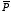perpendicular to its axis.
a) Find the electric field inside the cylinder.
b) Show that the field outside the cylinder can be expressed as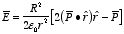### Problem 3

A thick spherical shell (inner radius a, outer radius b) is made of dielectric material with a "frozen-in" polarization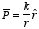where k is a constant and r is the distance from the center (see Figure 1).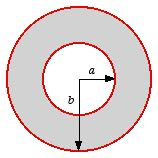Figure 1. Problem 3.
Find the electric field in all three regions by two different methods:
a) First calculate the bound charges and then calculate the field they produce.
b) Use Gauss's law to find the electric displacement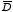, and then get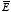.
Note: there is no free charge in the problem.

### Problem 4

A very long cylinder of linear dielectric material is placed in an otherwise uniform electric field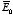. Find the resulting field within the cylinder. (The radius is R, the susceptibility χe, and the axis is perpendicular to.)

### Problem 5

Suppose you have enough linear dielectric material, of dielectric constant K, to half-fill a parallel-plate capacitor (see Figure 2).
a) By what fraction is the capacitance increased when you distribute the material as shown in Figure 2a?
b) By what fraction is the capacitance increased when you distribute the material as shown in Figure 2b?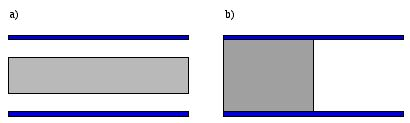Figure 2. Problem 5.